The General Linear Model# The General Linear Model

The General Linear Model (GLM) is mathematically identical to a multiple regression analysis but stresses its suitability for both multiple qualitative and multiple quantitative variables. The GLM is suited to implement any parametric statistical test with one dependent variable, including any factorial ANOVA design as well as designs with a mixture of qualitative and quantitative variables (covariance analysis, ANCOVA). Because of its flexibility to incorporate multiple quantitative and qualitative independent variables, the GLM has become the core tool for fNIRS data analysis after its introduction into the neuroimaging community by Friston and colleagues (Friston et al. 1994, 1995). The following sections briefly describe the mathematical background of the GLM in the context of fNIRS data analysis; a comprehensive treatment of the GLM can be found in the standard statistical literature, e.g., Draper and Smith (1998) and Kutner et al. (2005).

Note: In the fNIRS literature, the term “General Linear Model” refers to its univariate version. The term “univariate” does in this context not refer to the number of independent variables, but to the number of dependent variables. As mentioned earlier, a separate statistical analysis is performed for each channel time series (dependent variable). In its general form, the General Linear Model has been defined for multiple dependent variables, i.e., it encompasses tests as general as multivariate covariance analysis (MANCOVA).

From the perspective of multiple regression analysis, the GLM aims to “explain” or “predict” the variation of a dependent variable in terms of a linear combination (weighted sum) of several reference functions. The dependent variable corresponds to the observed fNIRS time course of a channel, and the reference functions correspond to time courses of expected (idealized) fNIRS responses for different conditions of the experimental paradigm. The reference functions are also called predictors, regressors, explanatory variables, covariates, or basis functions. A set of specified predictors forms the design matrix, also called the model. A predictor time course is typically obtained by a convolution of a condition box-car time course with a standard hemodynamic response function (two-gamma HRF or single-gamma HRF). A condition box-car time course may be defined by setting values to 1 at time points, at which the modeled condition is defined (“on”), and 0 at all other time points. Each predictor time course X gets an associated coefficient or beta weight b, quantifying its potential contribution in explaining the channel time course y. The channel time course y is modeled as the sum of the defined predictors, each multiplied with the associated beta weight b. Since this linear combination will not perfectly explain the data due to noise fluctuations, an error value e is added to the GLM system of equations with n data points and p predictors: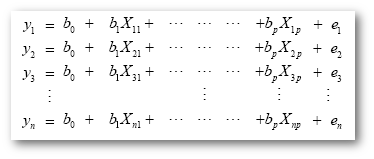The variable y on the left side corresponds to the data, i.e., the measured time course of a single channel. Time runs from top to bottom, i.e., y1 is the measured value at time point 1, y2 the measured value at time point 2 and so on. The channel time course (left column) is “explained” by the terms on the right side of the equation. The first column on the right side corresponds to the first beta weight b0. The corresponding predictor time course X0 has a value of 1 for each time point and is, thus, also called “constant”. Since multiplication with 1 does not alter the value of b0, this predictor time course (X0) does not explicitly appear in the equation. After estimation (see below), the value of b0 typically represents the signal level of the baseline condition and is also called intercept. While its absolute value is not informative, it is important to include the constant predictor in a design matrix, since it allows the other predictors to model small condition-related fluctuations as increases or decreases relative to the baseline signal level. The other predictors on the right side model the expected time courses of different conditions. For multi-factorial designs, predictors may be defined coding combinations of condition levels in order to estimate main and interaction effects. The beta weight of a condition predictor quantifies the contribution of its time course in explaining the channel time course. While the exact interpretation of beta values depends on the details of the design matrix, a large positive (negative) beta weight typically indicates that the channel exhibits strong activation (deactivation) during the modeled experimental condition relative to baseline. All beta values together characterize a channels’ “preference” for one or more experimental conditions. The last column in the system of equations contains error values, also called residuals, prediction errors, or noise. These error values quantify the deviation of the measured channel time course from the predicted time course, the linear combination of predictors.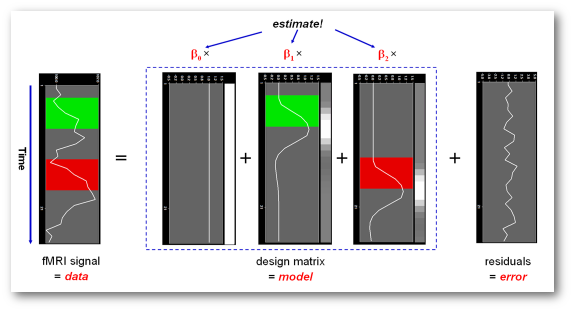The GLM system of equations may be expressed elegantly using matrix notation. For this purpose, we represent the channel time course, the beta values and the residuals as vectors, and the set of predictors as a matrix: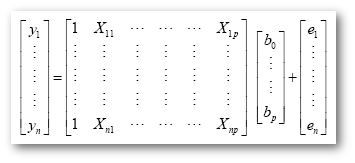Representing the indicated vectors and matrix with single letters, we obtain this simple form of the GLM system of equations: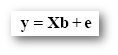In this notation, the matrix X represents the design matrix containing the predictor time courses as column vectors. The beta values now appear in a separate vector b. The term Xb indicates matrix-vector multiplication. The figure above shows a graphical representation of the GLM. Time courses of the signal, predictors and residuals have been arranged in column form with time running from top to bottom, as in the system of equations.

Given the data y and the design matrix X, the GLM fitting procedure has to find a set of beta values explaining the data as good as possible. The time course values predicted by the model are obtained by the linear combination of the predictors: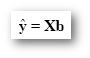A good fit would be achieved with beta values leading to predicted values, which are as close as possible to the measured values y. By rearranging the system of equations, it is evident that a good prediction of the data implies small error values: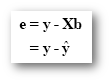An intuitive idea would be to find those beta values minimizing the sum of error values. Since the error values contain both positive and negative values (and because of additional statistical considerations), the GLM procedure does not estimate beta values minimizing the sum of error values, but finds those beta values minimizing the sum of squared error values: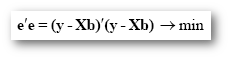The term e’e is the vector notation for the sum of squares (Sigma e2). The apostrophe symbol denotes transposition of a vector or matrix. The optimal beta weights minimizing the squared error values (the “least squares estimates”) are obtained non-iteratively by the following equation: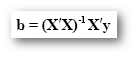The term in brackets contains a matrix-matrix multiplication of the transposed, X’, and non-transposed, X, design matrix. This term results in a square matrix with a number of rows and columns corresponding to the number of predictors. Each cell of the X’X matrix contains the scalar product of the two predictor vectors. The scalar product is obtained by summing all products of corresponding entries of two vectors corresponding to the calculation of covariance. This X’X matrix, thus, corresponds to the predictor variance-covariance matrix. The variance-covariance matrix is inverted, as denoted by the “-1” symbol. The resulting matrix (X’X)-1 plays an essential role not only for the calculation of beta values, but also for testing the significance of contrasts (see below). The remaining term on the right side, X’y, evaluates to a vector containing as many elements as predictors. Each element of this vector is the scalar product (covariance) of a predictor time course with the observed channel time course.

An interesting property of the least squares estimation method is that the variance of the measured time course can be decomposed into the sum of the variance of the predicted values (model-related variance) and the variance of the residuals: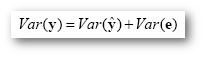Since the variance of the channel time course is fixed, minimizing the error variance by least squares corresponds to maximizing the variance of the values explained by the model. The square of the multiple correlation coefficient R provides a measure of the proportion of the variance of the data, which can be explained by the model: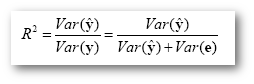The values of the multiple correlation coefficient vary between 0 (no variance explained) to 1 (all variance explained by the model). A coefficient of R = 0.7, for example, corresponds to an explained variance of 49% (0.7x0.7). An alternative way to calculate the multiple correlation coefficient consists in computing a standard correlation coefficient between the predicted values and the observed values: R = ryy. This equation offers another view on the meaning of the multiple correlation coefficient quantifying the interrelationship (correlation) of the combined set of predictor variables with the observed time course.

NOTE: The R2 can be displayed for HbO and Hb data if you hover with the mouse over the respective channel HbO or Hb cell in the channel selection table, as shown below:## GLM Diagnostics

Note that if the design matrix (model) does not contain all relevant predictors, condition-related increases or decreases in the channel time course will be explained by the error values instead of the model. It is, therefore, important that the design matrix is constructed with all expected effects, which may also include reference functions not related to experimental conditions, for example, estimated motion parameters or drift predictors, if not removed during preprocessing. When all expected effects are properly modeled, the residuals should reflect only noise fluctuations. If some effects are not (correctly) modeled, a plot of the residuals will show low frequency fluctuations instead of a stationary noisy time course. A visualization of the residuals is, thus, a good diagnostic to assess whether the design matrix has been defined properly.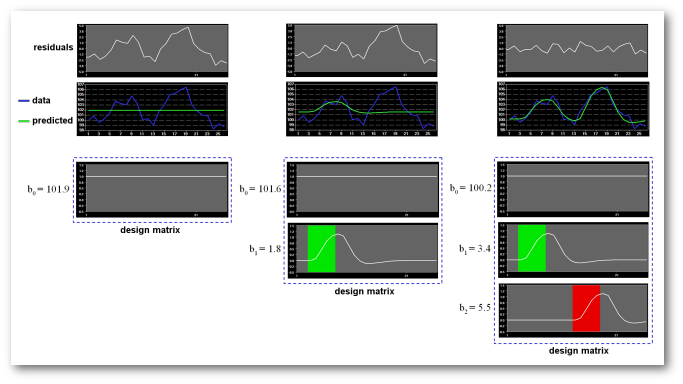Note: To inspect the added predicted signal and the residuals you can navigate to the Analysis menu and select the GLM Diagnostics entry, as shown below.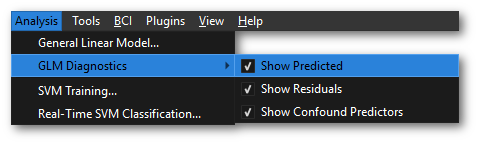Here you can select to show the Predicted using the Show Predicted option, or the Residuals time course using the Show Residuals option. These will be visualized in the selected channel plot (top left).

## GLM Significance Tests

The multiple correlation coefficient is an important measure of the “goodness of fit” of a GLM. In order to test whether a specified model significantly explains variance in a channel time course, the R value can be transformed in an F statistic with p - 1 degrees of freedom in the numerator and n - p degrees of freedom in the denominator: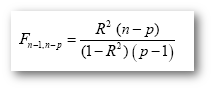With the known degrees of freedom, an F test can be converted in an error probability value p. A high F value (low p value) indicates that one or more experimental conditions substantially modulate the data time course. While the overall F statistic may tell us whether the specified model significantly explains a channel’s time course, it does not allow to asses which individual conditions differ significantly from each other. Comparisons between conditions can be formulated as contrasts, which are linear combinations of beta values corresponding to null hypotheses. To test, for example, whether activation in a single condition i deviates significantly from baseline, the null hypothesis would be H0: b1 = 0. To test whether activation in condition 1 is significantly different from activation in condition 2, the null hypothesis would state that the beta values of the two conditions would not differ, H0: b1 = b2 or H0: (+1)b1 + (-1)b2 = 0. To test whether the mean of condition 1 and condition 2 differs from condition 3, the following contrast could be specified: H0: (b1 + b2)/2 = b3 or H0: (+1)b1 + (+1)b2 + (-2)b3 = 0. The values used to multiply the respective beta values are often written as a contrast vector c. In the latter example, the contrast vector would be written as c = [+1 +1 -2]. Note that the constant term is treated as a confound and it is not included in contrast vectors, i.e., it is implicitly assumed that b0 is multiplied by 0 in all contrasts. To include the constant explicitly, each contrast vector must be expanded by one entry at the beginning or end. Using matrix notation, the linear combination defining a contrast can be written as the scalar product of contrast vector c and beta vector b. The null hypothesis can then be simply described as c’b = 0. For any number of predictors k, such a contrast can be tested with the following t statistic with n - p degrees of freedom: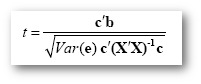The numerator of this equation contains the described scalar product of the contrast and beta vector. The denominator defines the standard error of c’b, i.e., the variability of the estimate due to noise fluctuations. The standard error depends on the variance of the residuals Var(e), as well as on the design matrix X, but not on the data time course y. With the known degrees of freedom, a t value for a specific contrast can be converted in an error probability value p using the equation shown earlier. Note that the null hypotheses above were formulated as c’b = 0, implying a two-sided alternative hypothesis, i.e., Ha: c’b != 0. A two-sided test is used as default in Turbo-Satori. For one-sided alternative hypotheses, e.g., Ha: b1 > b2, the obtained p value from a two-sided test can be simply divided by 2 to get the p value for the one-sided test. If this p value is smaller than 0.05 and if the t value is positive, the alternative hypothesis may be concluded.

## Conjunction Analysis

Experimental research questions often lead to specific hypotheses, which can best be tested by the conjunction of two or more contrasts. As an example, it might be interesting to test with contrast c1 whether condition 2 leads to significantly higher activity than condition 1, and with contrast c2 whether condition 3 leads to significantly higher activity than condition 2. This question could be tested with the following conjunction contrast: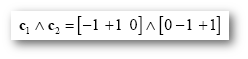Note that a logical “and” operation is defined for Boolean values (true / false), but that t values associated with individual contrasts can assume any real value. An appropriate way to implement a logical “and” operation for conjunctions of contrasts with continuous statistical values is to use a minimum operation, i.e., the significance level of the conjunction contrast is set identical to the significance level of the contrast with the smallest t value: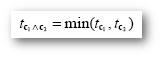## Multicollinear Design Matrices

Multicollinearity exists when predictors of the design matrix are highly intercorrelated. To assess multicollinearity, pairwise correlations between predictors are not sufficient. A better way to detect multicollinearity is to regress each predictor variable on all the other predictor variables and examine the resulting R2 values. Perfect or total multicollinearity occurs when a predictor of the design matrix is a linear function of one or more other predictors, i.e., when predictors are linearly dependent on each other. While in this case solutions for the GLM system of equations still exist, there is no unique solution for the beta values. From a mathematical perspective of the GLM, the square matrix X’X becomes singular, i.e. it loses (at least) one dimension, and is no longer invertible in case that X exhibits perfect multicollinearity. Matrix inversion is required to calculate the essential term (X’X)-1 used for computing beta values and standard error values (see above). Fortunately, special methods, including singular value decomposition (SVD), allow obtaining (pseudo-) inverses for singular (rank deficient) matrices. Note, however, that in this case the absolute values of beta weights are not interpretable and statistical hypothesis tests must meet special restrictions. In fNIRS design matrices, multicollinearity occurs if all conditions are modeled as predictors in the design matrix, including the baseline (rest, control) condition. Without the baseline condition, multicollinearity is avoided, and beta weights are obtained, which are easily interpretable. As an example, consider the case of two main conditions and a rest condition. If we would not include the rest condition (recommended), the design matrix would not be multicollinear and the two beta weights b1 and b2 would be interpretable as increase or decrease of activity relative to the baseline signal level modeled by the constant term (see figure above, right column). This approach is used in Turbo-Satori and is especially important when running GLMs for random-effects group analyses. Contrasts could be specified to test single beta weights, e.g., the contrast c = [1 0] would test whether condition 1 leads to significant (de)activation relative to baseline. Furthermore, the two main conditions could be compared with the contrast c =[-1 1], which would test whether condition 2 leads to significantly more activation than condition 1. If we created the design matrix including a predictor for the rest condition, we would obtain perfect multicollinearity and the matrix X’X would be singular. Using a pseudo-inverse or SVD approach, we would now obtain three beta values (plus the constant), one for the rest condition, one for main condition 1 and one for main condition 2. While the values of beta weights might not be interpretable, correct inferences of contrasts can be obtained, if an additional restriction is met; typically, that the sum of the contrast coefficients equals 0. To test whether main condition 1 differs significantly from the rest condition, the contrast c = [-1 +1 0] would now be used. The contrast c = [0 -1 +1] would be used to test whether condition 2 leads to more activation than condition 1.

## GLM Assumptions

Given a correct model, the estimation routine (ordinary least squares, OLS) of the GLM operates correctly only under the following assumptions. The population error values ε must have an expected value of zero at each time point, i.e. E[εi] = 0, and constant variance, i.e., Var[εi] = σ2. Furthermore, the error values are assumed to be uncorrelated, i.e. Cov(εij) = 0 for all i != j. To justify the use of t and F distributions in hypothesis tests, errors are further assumed to be normally distributed, i.e., ei ~ N(0, σ2). In summary, errors are assumed to be normal, independent and identically distributed (abbreviated as “normal i.i.d.”). Under these conditions, the solution obtained by the least squares method is optimal in the sense that it provides the most efficient unbiased estimation of the beta values. While robust to small violations, assumptions should be checked using sample data. For fNIRS measurements, the assumption of uncorrelated error values requires special attention.

## Correction for serial correlations

To ensure accuracy of the GLM for the analysis of fNIRS data, global assumptions of model and noise need to be fullfilled. The GLM, as shown in formula below, consists of basically three elements: the fNIRS time series Y, the design matrix X incorporating the regressors modeling the brain responses and signal confounds, and the β weights which are the parameters to be estimated and the residuals term ɛ.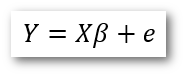One assumption for the residuals of the GLM, the noise, is that it is uncorrelated. But it has been already shown for fNIRS data that this assumption is not met. There are different sources causing the temporal correlation in fNIRS, e.g., trends or physiological noise. To overcome this problem the fMRI time series needs to be preprocessed to remove these correlations. Here we only focus on one of the most common processes, the pre-whitening, which should also perform best in block based stimuli paradigms. To whiten the residuals, multiple steps need to be performed and different models can be used for the pre-whitening method, such as auto-regressive models (AR), moving-average (MA), or auto-regressive-moving-average. As the AR model is very often used in fNIRS analysis, we decided to use this method.

First an auto-regressive model is estimated for each channel separately. For order AR(1), this can be done by estimating the amount of serial correlation r in the residuals using pairs of successive residual values (εtt+1), i.e., the residual time course is correlated with itself shifted by one-timepoint (lag = 1). In the second step, the estimated serial correlation is removed from the measured channel time course by calculating the transformed time course: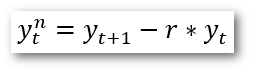The superscript “n” indicates the values of the new, adjusted time course. While the AR(1) method for removal of serial correlations works well for fNIRS with low repetition time (e.g., 2 seconds), it has been shown that a second-order, AR(2), model outperforms the AR(1), as well as other approaches used in fNIRS data analysis. The AR model of a higher order p can be described as: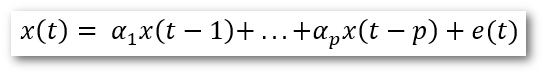The parameter e(t) is the white noise, and parameters α_1,…,α_p are the AR parameters to be estimated for the different model orders (Eklund et al., n.d.). A model order needs to be selected objectively. This can be done using different information criteria. The main task is to minimize the information criteria function. There are multiple information criteria available which were compared in (Liew, n.d.), for example Akaike information criterion, Schwarz information criterion, Hannan-Quinn criterion and the Bayesian information criterion.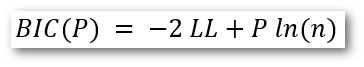All criteria become more accurate the higher the sample size is. Because BIC was already used in fNIRS analysis and showed good results, this criterion was also used in TSI.

The BIC consists of the following parts: P is the model order; LL is the log-likelihood of the model fit, and n is the number of time points.

After the selection of the AR(p) order, the AR parameters need to be estimated using Burg’s maximum entropy method.

Although very often used, we decided not to use the Yule-Walker approach, because it may lead to incorrect parameter estimates in case of nearly periodic signals. Instead, we used the best alternative, Burg’s method. “The Burg estimator is a close relative of the Yule–Walker estimator. It entails a recursive algorithm which is aimed at finding the sequence of values which constitutes the (empirical) partial auto-correlation function and which is also described as reflection coefficients” (Pollock, 1999). The estimated AR parameters are then used to whiten the time series and model predictors using the following equation: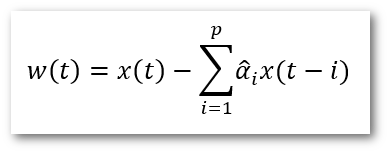Here p is the order of the AR model and αi are the model parameter estimates. After the pre-whitening, the GLM is performed again, using the new time series and model predictors.